# DAV Class 7 Maths Chapter 9 Worksheet 4 Solutions

The DAV Maths Class 7 Solutions and DAV Class 7 Maths Chapter 9 Worksheet 4 Solutions of Construction of Triangles offer comprehensive answers to textbook questions.

## DAV Class 7 Maths Ch 9 WS 4 Solutions

Question 1.
∆ ABC is isosceles with AB = AC. AD is the altitude from A on BC.(i) Is ∆ ABD ≅ ∆ ACD? Why?
(ii) State the three pairs of matching parts you have used to answer (i)
(iii) Is it true to say that BD = CD? Why?
In ∆ ABD and ∆ ACD,
AB = AC (given)
(i) ∴ ∆ ABD ≅ ∆ ACD (RHS)
(iii) BD = CD [Corresponding parts of congruent triangles]Question 2.
In the given figure, which pairs of triangles are congruent by RHS congruence condition? If congruent, write the result in symbolic form.In ∆ ADB and ∆ ACB,
∠ADB = ∠ACB = 90° (given)
AD = BC = 3 cm (given)
hypt. AB = hypt. AB (common)
∆ ADB ≅ ∆ ACB (By RHS)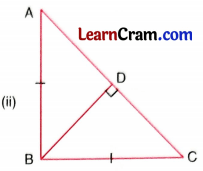In ∆ BDC and ∆ ADB
hypt.BC = hypt. AB (given)
∠BDC = ∠ADB – 90° (given)
BD = BD (common)
∆ BDC ≅ ∆ ADB (By RHS)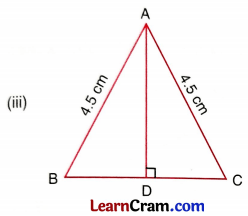AB = AC = 4.5 cm (given)∠ABC = ∠ADC = 90° (given)
BC = DC = 6.4 cm (given)
AC = AC (common)
∆ ABC ≅ ∆ ADC (By RHS)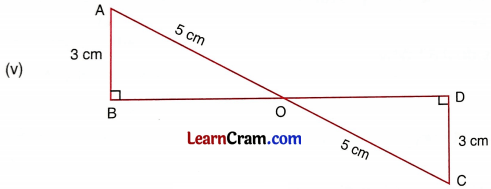AB = CD = 3 cm (given)
∠ABO = ∠CDO = 90° (given)
OA = OC = 5 cm (given)
∆ ABO ≅ ∆ CDO (By RHS)

Question 3.
QS and RT are the altitudes of ∆PQR, and QS = RT (See fig.).(i) Is ∆ QRS ≅ ∆ RQT by RHS congruence condition ?
In ∆ QRS and ∆ RQT,
QS = RT (given)
∠QTR = ∠RSQ = 90° (given)
QR = QR (Common)
∆QRS ≅ ∆RQT (By RHS)

(ii) State the three pairs of corresponding parts which make ∆ QRS ≅ ∆ RQT.
QS and RT, ∠QTR and ∠RSQ,
QR = QRQuestion 4.
In the given figure, ∠B = ∠P = 90° and side AB = side PR. What additional information is required to make ∆ABC ≅ ∆RPQ by RHS congruence condition?In ∆ABC and ∆PQR,
AB = PR = 4 cm (given)
∠ABC = ∠QPR = 90° (given)
AC = QR (is required)
Hence AC = QR is required information to make ∆ABC ≅ ∆RPQ by RHS congruence condition.

Question 5.
In the given figure, AC = DB and AB ⊥ BC. Also DC ⊥ BC.State which of the following statements is true.
(i) ∆ABC ≅ ∆DBC
(ii) ∆ ABC ≅ ∆DCB
(iii) ∆ ABC ≅ ∆ BCD
State the three pairs of matching parts you have used to arrive at the answer. Which side is equal to side AB? Why?
In ∆ABC and ∆DCB,
(i) AC = DB
∠ABC = ∠DCB = 90°
BC = BC
∆ABC ≅ ∆DCB
Hence (ii) statement is true.
Matching parts are
AC and DB, ∠ABC and ∠DCB, BC and BC
AB = DC [Corresponding parts of congruent triangles are equal]

### DAV Class 7 Maths Chapter 9 Value Based Questions

Question 1.
Namita got a chocolate on her friend’s birthday from school. She took it home and cut into two congruent triangles. She gave one piece to her maid’s daughter Meenu and had the other piece herself. You are given the way in which Namita divided the chocolate.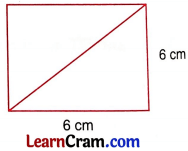(i) What congruence criteria did Namita use here?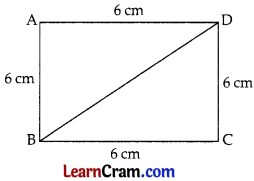Let ABCD be a chocolate. Namita cut it into two congruent triangles along diagonal BD.
So, the two triangles are AABD and ACBD we have
AB = CD = 6 cm [From the figure]
BC = AD = 6 cm [Fromg the figure]
BD = BD [Common]
So, by SSS criterion of congruence,
∆ABD ≅ ∆CBD
Hence, Namita used SSS criterion of congruence.

(ii) What value of Namita is depicted here?
Solution:
Values: Sharing things equally among the friends or people.
Helping the poor.Question 2.
Aditi wanted to make a crown for her sister who was going to act as a queen in a school programme. She found a used cardboard of length 24 cm and breadth 20 cm. She drew two congruent triangles after marking the mid-point of length as X.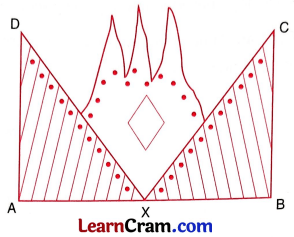Then she decorated the crown with beads and stars.
Her sister was very happy to see the beautiful crown.
(i) What congruence criteria did Aditi use here?Here, length of the cardboard AB = 24 cm.
As X is the mid-point of AB, therefore,
AX = XB = y (AB)
⇒ AX = XB = 12 cm
And, the breadth of the cardboard = 20 cm.
So, from the figure, AD = 20 cm and BC = 20 cm.
Now, in ∆DAX and ∆CBX, we have
AX = XB = 12 cm
AD = BC = 20 cm
∠DAX = ∠CBX
So, by SAS criterion of congruence,
∆DAX ≅ ∆CBX
Hence, Aditi used SAS criterion of congruence here.

(ii) What value of Aditi is depicted here?